# Find the unit vectors perpendicular to the given vectors

• brochesspro
Which means that the equation has been solved for ##a##.No, the following would not be another equation in the variables a, b and c due to the fact that the unknown vector is a unit vector. If yes, then we end up with 3 equations for the three variables, which now can be solved for a, b and c.$$a^2 + b^2 + c^2= 1$$We could find ##a## in terms of ##b## from first equation. Then from second equation, substituting ##a## in...$$\vec{a} = \frac{1}{bf #### brochesspro Homework Statement Given below. Relevant Equations Given below.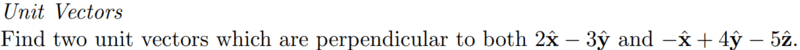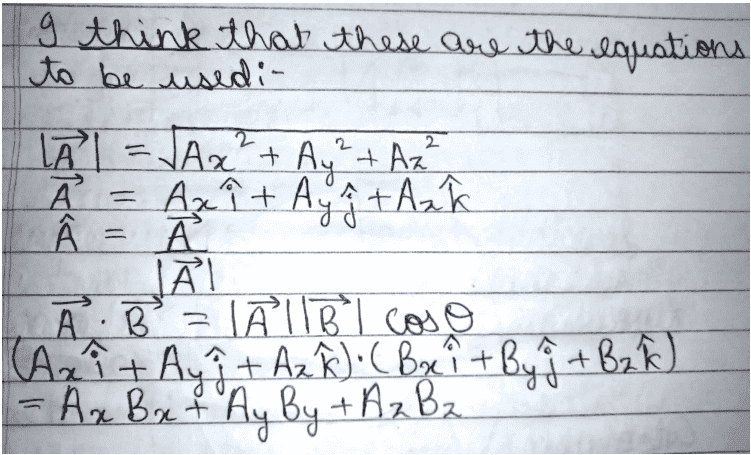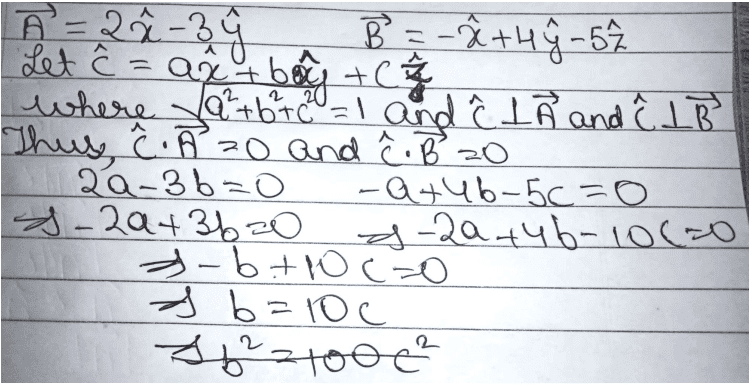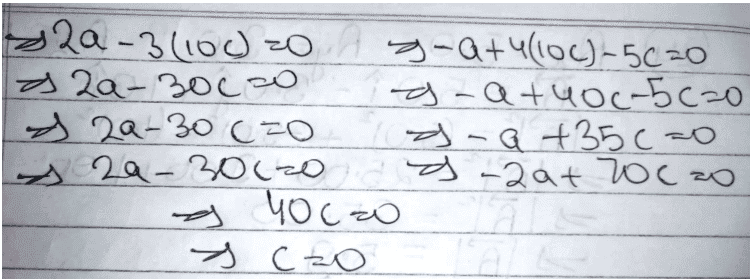Check the equation beneath ##-a+4b-5c=0##. :) (can you solve it faster another way? what are the properties of the cross product?) •PhDeezNutz Yeah I’m going to have to echo the comment about using cross products. Take the cross product of the two vectors and find its unit vector. Then negate it and you have your two answers. •vcsharp2003 (can you solve it faster another way? what are the properties of the cross product?) Do you mean dot product? Do you mean dot product?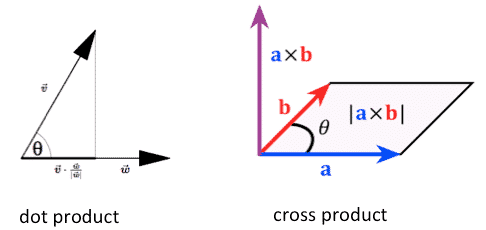•PeroK Do you mean dot product? I think they meant the cross product! •PhDeezNutz Yeah I’m going to have to echo the comment about using cross products. Take the cross product of the two vectors and find its unit vector. Then negate it and you have your two answers. You gave me the answer too soon. It would have been better if you would have slowly led me to that conclusion. I think they meant the cross product! I was only focusing on the perpendicular part and the fact that perpendicular vectors have dot product 0. And so, I completely forgot about cross products. But still, thank you for the responses. Now I can solve this question on my own. You gave me the answer too soon. It would have been better if you would have slowly led me to that conclusion. Fair enough. Let’s back track. Can you prove that. Let’s justify this approach. ##(\vec{a} \times \vec{b}) \cdot \vec{b} = (\vec{a} \times \vec{b}) \cdot \vec{a} = 0##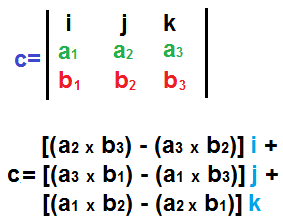I was only focusing on the perpendicular part and the fact that perpendicular vectors have dot product 0. And so, I completely forgot about cross products. That's a good point. You could look generate simultanenous equations by looking for a vector whose dot product with both those vectors is zero. Using the cross product could be quicker. Fair enough. Let’s back track. Can you prove that. Let’s justify this approach. ##(\vec{a} \times \vec{b}) \cdot \vec{b} = (\vec{a} \times \vec{b}) \cdot \vec{a} = 0## View attachment 295004 No need for it now, I already got the answer. But, thank you. That's a good point. You could look generate simultanenous equations by looking for a vector whose dot product with both those vectors is zero. Using the cross product could be quicker. I tried to do that itself. Could you once go through the attachments and guide me to what I have done wrong? I tried to do that itself. Could you once go through the attachments and guide me to what I have done wrong? Never mind, I got the answer. Again, thank you for all your responses. I tried to do that itself. Could you once go through the attachments and guide me to what I have done wrong? You got as far as$$2a -3b = 0-a + 4b - 5c =0$$And then things seemed to go a bit wrong. I just multiplied the second equation by ##2## and then added them. Note that you are always going to have a free parameter here. In this case ##c## can be anything. PS I like to write vectors as ##(2, -3, 0), (-1, 4, -5), (a, b, c)##. Has this gone out of fashion? Anyway, it seems very simple to write down the dot products in this way. PS I like to write vectors as ##(2, -3, 0), (-1, 4, -5), (a, b, c)##. Has this gone out of fashion? Anyway, it seems very simple to write down the dot products in this way. I am not sure about it. But, many people write it that way, I think it is used for points rather than vectors, but I may be wrong. You got as far as$$2a -3b = 0-a + 4b - 5c =0$$And then things seemed to go a bit wrong. I just multiplied the second equation by ##2## and then added them. Note that you are always going to have a free parameter here. In this case ##c## can be anything. Yes, I noticed that I forgot to multiply the 4 by 2 and things went downhill from there. But, I can now do this both ways, the dot product way, and the cross product way. Goodbye for now. •PhDeezNutz and PeroK You got as far as$$2a -3b = 0-a + 4b - 5c =0$$And then things seemed to go a bit wrong. I just multiplied the second equation by ##2## and then added them. Note that you are always going to have a free parameter here. In this case ##c## can be anything. Wouldn't the following be another equation in the variables a, b and c due to the fact that the unknown vector is a unit vector? If yes, then we end up with 3 equations for the three variables, which now can be solved for a, b and c.$$a^2 + b^2 + c^2= 1$$We could find ##a## in terms of ##b## from first equation. Then from second equation, substituting ##a## in terms of ##b##, we will get ##c## in terms of ##b##. Now, we substitute in above equation the variables ##a## and ##c##, and solve for ##b##. Knowing ##b## means we can determine ##a## and ##c##. Last edited: •PhDeezNutz Wouldn't the following be another equation in the variables a, b and c due to the fact that the unknown vector is a unit vector? If yes, then we end up with 3 equations for the three variables, which now can be solved for a, b and c.$$a^2 + b^2 + c^2= 1We could find ##a## in terms of ##b## from first equation. Then from second equation, substituting ##a## in terms of ##b##, we will get ##c## in terms of ##b##. Now, we substitute in above equation the variables ##a## and ##c##, and solve for ##b##. Knowing ##b## means we can determine ##a## and ##c##.
That is what I did, but with ##c##.

That is what I did, but with ##c##.
That should give you two different unit vectors.

Yes, I got them.